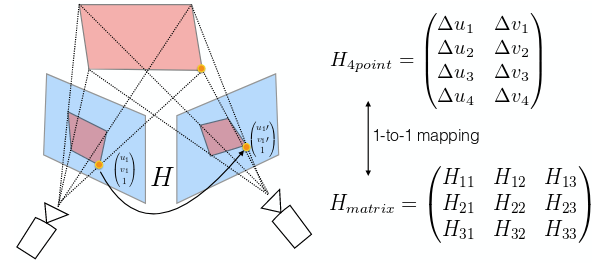# How to transform 4 points parameter matrix to homography matrix

As the title say, how could I transform 4 points parameter matrix to homography matrix?

The 4 points parameter matrix storing the delta of 4 corresponding points

``````delta_x1 = x1_b - x1_a
delta_y1 = y1_b - y1_a
.
.
.
.
delta_x4 = x4_b - x4_a
delta_y4 = y4_b - y4_a
``````Please tell me if my questions are unclear, thanks

I ask this because I have trained a model based on the paper–Deep image homography estimiation, results looks very great on train/test set.

I generate the test set with the same solution introduced by the paper, but instead of generate images with size 256256, I generate the images with size 128128.

After that, I measure the loss as the sqrt of l2 loss

``````for loop:
real_delta = points[rng]
name_list = imgs_names[rng]
results = sess.run(_accuracy, feed_dict = {_features: read_imgs(name_list, imgs_folder, [None, 128, 128, 2])})

delta_diff = np.sum(np.abs(results - real_delta))
total_delta_diff += delta_diff

l2_loss = np.sum((results-real_points) ** 2)/2
sqrt_l2_loss = np.sqrt(l2_loss)
total_l2_loss += l2_loss
total_sqrt_l2_loss += sqrt_l2_loss
print("{},sqrt_l2_loss:{}, l2_loss:{}".format(i, sqrt_l2_loss, l2_loss))
print("{},delta diff:{}".format(i, delta_diff))

print('avg total l2 loss:{}, avg total sqrt l2 loss:{}'.format(total_l2_loss/iteration/batch_size, total_sqrt_l2_loss/iteration/batch_size))
print("avg delta diff:{}".format(total_delta_diff/iteration/8.0/batch_size))
``````

avg sqrt L2 loss of training set(500032 images from image net) : 0.8967
avg sqrt L2 loss of test set(10000 images from image net) : 0.8448
avg delta of test set(how many pixels different with the real delta) : 2.48

Dear Tham,

have you figured it out? I am facing the same issue.

Thank you
Giovanni

This link might be a good starting point: https://math.stackexchange.com/questions/494238/how-to-compute-homography-matrix-h-from-corresponding-points-2d-2d-planar-homog.

Also OpenCV (C++ or Python) should have the needed function. Check out this post: https://www.learnopencv.com/homography-examples-using-opencv-python-c/

1 Like

I read those articles previously. The problem for me is that when I receive the output from the trained system I only have the 4 point parametrization matrix and I dont know any point in the image I am trying to discover the homograpy matrix. Maybe I would have to infer 4 points? I am not quite sureMaybe I didn’t understand correctly. From your model, you have the parametrization matrix. Is this correct? If so, then what are you looking for after this?

1 Like

I did the process as follows:

1. I choose 4 points on image A and the 4 correspondent points in the image B
2. I generated the H4point matrix ( delta_x1 = x1_b - x1_a, delta_y1 = y1_b - y1_a … )
3. Then I trained all my model with those images and this 4 point matrix;
4. When I predict over an new image the output is an H4point matrix and here is where I am stucked at;

Now I am trying to discover how I get the H_matrix (3x3 matrix) starting from the H4point.I believe you use the 4 points in the matrix( they are 4 pairs of points) as input into the opencv program getPerspectivetransform(). IFrom the paper, it looks like this is what the authors did. Check this out: https://opencv-python-tutroals.readthedocs.io/en/latest/py_tutorials/py_imgproc/py_geometric_transformations/py_geometric_transformations.html

1 Like

First I tought it would be as simple as this, but no it is not. The output is composed by the deltas. The only way I see I could start from deltas and get te original points is if I already knew the distance bettwen them, but this is not true. Event though, assuming I know that, it would be a little complex to get the correct points.

I am really stucked. I am thinking about proportions, but I guess this is not the answer too.

Would this work: The output of the model is H_delta_estimated ~ [u_orig - u_transformed].

The input of the model is known, u_orig. Then: u_transformed_estimated = u_orig - H_delta_estimated.

To get H_estimated, then you should be able to do this:
H_estimated = cv2. getPerspectiveTransform(u_orig, u_transformed_estimated) .

I have not had a chance to code this up but the logic should work. What do you think?

Hi nchukaobah,
but what is u_orig? the corners of original image?